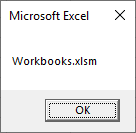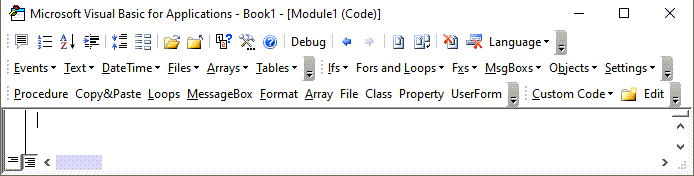# VBA ActiveWorkbook vs. ThisWorkbook

This tutorial will discuss the difference between the VBA ActiveWorkbook and ThisWorkbook objects.

## ActiveWorkbook vs. ThisWorkbook

It’s important to the know the difference between the ActiveWorkbook and ThisWorkbook in VBA:

The ActiveWorkbook is the workbook that is currently active (similar to how ActiveSheet is the currently active sheet).  ThisWorkbook is the workbook where the VBA code is stored.  ThisWorkbook will never change.

### ThisWorkbook

Think of ThisWorkbook as an object variable that allows you to reference the workbook containing the currently running code.

This code will display a MessageBox with ThisWorkbook name:

``````Sub Show_ThisWorkbook()
MsgBox ThisWorkbook.Name
End Sub``````### ActiveWorkbook

The ActiveWorkbook is an object variable that allows you to reference the currently active workbook.

This code will display a MessageBox with ActiveWorkbook name:

``````Sub Show_ActiveWorkbook()
MsgBox ActiveWorkbook.Name
End Sub``````

## VBA Assumes ActiveWorkbook

When attempting to work with objects (ex. Sheets) within the ActiveWorkbook, you do not need to explicitly state the ActiveWorkbook object. VBA will assume you are referring to the ActiveWorkbook.

So this:

``ActiveWorkbook.Sheets("Sheet1").Range("\$A\$5").Value = 1``

Is the same as this:

``Sheets("Sheet1").Range("\$A\$5").Value = 1``

## New or Opened Workbooks are Active

Whenever you create a new workbook or open a workbook, the workbook becomes “Active”. You can see for yourself with this code that will add a workbook and retrieve the new workbook’s name:

``````Sub Show_ActiveWorkbook_Add()
MsgBox ActiveWorkbook.Name
End Sub``````

After adding or opening a workbook, you can assign it to a variable by using the ActiveWorkbook object. We will show you how in the examples below:

## ThisWorkbook and ActiveWorkbook Examples

Stop searching for VBA code online. Learn more about AutoMacro - A VBA Code Builder that allows beginners to code procedures from scratch with minimal coding knowledge and with many time-saving features for all users!### Switch Active Workbook

Switch the active workbook using Workbook name:

``Workbooks("Book1").Activate``

Switch the active workbook using an index as a workbook order number (1 is the first workbook opened or created):

``Workbooks(1).Activate``

### Make ThisWorkbook Active

Make ThisWorkbook (where the currently running code is stored) the ActiveWorkbook:

``ThisWorkbook.Activate``

### Set ActiveWorkbook to a Variable

Assign the ActiveWorkbook to a workbook object variable:

``````Dim wb As Workbook
Set wb = ActiveWorkbook``````

VBA Programming | Code Generator does work for you!

### Close and Save the Active Workbook

Closes and Saves the ActiveWorkbook:

``ActiveWorkbook.Close SaveChanges:=True``

### Close the Active Workbook Without Saving

Closes the ActiveWorkbook without saving:

``ActiveWorkbook.Close SaveChanges:=False``

### ActiveWorkbook – Save As

Performs a Save As of the active Workbook.

``````Sub SaveAsActiveWorkbook()
Dim result As Variant
result = Application.GetSaveAsFilename(InitialFileName:="", _
FileFilter:="Excel Macro-Enabled Workbook (*.xlsm), *.xlsm,Excel Workbook (*.xlsx), *.xlsx")

If result = False Then Exit Sub

ActiveWorkbook.SaveAs result
End Sub
``````